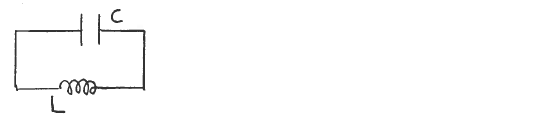# Problem: A charged capacitor with C = 4.00 x 10 -12 F and initial charge Q = 8.00 x 10 -6 C is connected to an inductor that has L = 4.0 H. During the subsequent current oscillations, what is the maximum current in the inductor? A) 2.0 A B) 4.0 A C) 6.0 A D) 8.0 A E) None of the above answers

###### FREE Expert Solution
90% (218 ratings)
###### Problem Details

A charged capacitor with C = 4.00 x 10 -12 F and initial charge Q = 8.00 x 10 -6 C is connected to an inductor that has L = 4.0 H. During the subsequent current oscillations, what is the maximum current in the inductor?

A) 2.0 A

B) 4.0 A

C) 6.0 A

D) 8.0 A

E) None of the above answersFrequently Asked Questions

What scientific concept do you need to know in order to solve this problem?

Our tutors have indicated that to solve this problem you will need to apply the LC Circuits concept. You can view video lessons to learn LC Circuits. Or if you need more LC Circuits practice, you can also practice LC Circuits practice problems.

How long does this problem take to solve?

Our expert Physics tutor, Juan took 2 minutes and 59 seconds to solve this problem. You can follow their steps in the video explanation above.